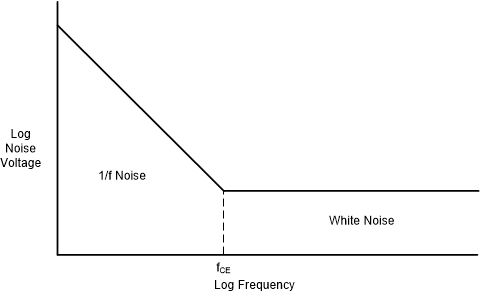SLOA011B January   2018  – July 2021

1. 1Introduction
2. 2Non-Inverting Amplifier
3. 3Inverting Amplifier
4. 4Simplified Op Amp Circuit Diagram
5. 5Op Amp Specifications
6. 6References
7. 7Glossary
8. 8Revision History

## 5.14 Equivalent Input Noise

All op amps have associated parasitic noise sources. Noise is measured at the output of an op amp and referenced back to the input; thus, it is called equivalent input noise.

Equivalent input noise specifications are usually given in two ways. One way is to specify the spot noise; that is, the equivalent input noise is given as voltage, Vn, (or current, In) per root hertz at a specific frequency. The second way is to specify noise as a peak-to-peak value over a frequency band. A brief review of noise characteristics is necessary to explain these parameters.

The spectral density of noise in op amps has a 1/f and a white noise component. 1/f noise is inversely proportional to frequency and is usually only significant at low frequencies. White noise is spectrally flat. Figure 5-9 shows a typical graph of op amp equivalent input noise.

Usually spot noise is specified at two frequencies. The first frequency is usually 10 Hz, where the noise exhibits 1/f spectral density. The second frequency is typically 1 kHz, where the noise is spectrally flat. The units used are normally RMS nV√Hz (or RMS pA√Hz for current noise). In Figure 5-9 the transition between 1/f and white is denoted as the corner frequency, fCE.

A noise specification, such as VN(PP), is the a peak to peak voltage over a specific frequency band, typically 0.1 Hz to 1 Hz or 0.1 Hz to 10 Hz. The units of measurement are typically nV pk-pk. To convert noise voltages given in RMS to pk-pk, a factor around 6 is typically used to account for the high crest factor seen in noise voltages e.g. VN(PP) = 6 x VN(RMS.

Given the same structure within an op amp, increasing bias currents lowers noise (and increases SR, GBW, and power dissipation).

Also the resistance seen at the input to an op amp adds noise. Balancing the input resistance on the non-inverting input to that seen at the inverting input, while helping with offsets due to input bias current, adds noise to the circuit.Figure 5-9 Typical Op Amp Input Noise Spectrum